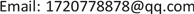1华北理工大学经济学院金融学专业，河北 唐山

2华北理工大学理学院数学与应用数学专业，河北 唐山1. 引言

2. 收得率预测评判标准

2.1. BP神经网络的C、Mn收得率预测模型建立

BP神经网络是一种非线性动力系统，包含输入层、隐含层、输出层三层单元。设输入向量为 X = ( x 1 , x 2 , ⋯ , x n ) T ，隐含层输出向量为 Y = ( y 1 , y 2 , ⋯ , y m ) T ，输出层输出向量为 G = ( g 1 , g 2 , ⋯ , g k ) T ，则有期望输出向量为 D = ( d 1 , d 2 , ⋯ , d l ) T ，输出层到隐含层之间的权值矩阵用V表示， V = ( v 1 , v 2 , ⋯ , v m ) ，其中列向量 v m 为隐含层第m个神经元对应的权向量，隐含层到输出层之间的权值矩阵用W表示， W = ( w 1 , w 2 , ⋯ , w i ) ，其中列向量 w i 为输出层第i个神经元对应的权向量 。

{ G j = f ( n e t j ) , j = 1 , 2 , ⋯ , k ,   输 入 层 n e t j = ∑ i = 0 m ω i j g i , j = 1 , 2 , ⋯ , k ,   隐 含 层 f ( x ) = 1 1 + e − x ,   转 移 函 数 f ′ ( x ) = f ( x ) [ 1 − f ( x ) ]

E = 1 2 ( D − G ) 2 = 1 2 ∑ i = 1 k ( d i − g i ) 2 (1)

E = 1 2 ∑ i = 1 k [ d i − f ( n e t i ) ] 2 = 1 2 ∑ i = 1 k [ d i − f ( n e t i = ∑ i = 0 m w i j g i ) ] 2 (2)

E = 1 2 ∑ i = 1 k { d i − f [ ∑ i = 0 m w i j f ( n e t i ) ] } 2 = 1 2 ∑ i = 1 k { d i − f [ ∑ i = 0 m w i j f ( ∑ i = 0 n v i j g i ) ] } 2 (3)

Δ w i j = − η ∂ E ∂ w i j , i = 0 , 1 , 2 , ⋯ , n ; j = 1 , 2 , ⋯ , k Δ v m i = − η ∂ E ∂ v m i , m = 0 , 1 , 2 , ⋯ , l ; i = 1 , 2 , ⋯ , n (4)

2.2. BP神经网络的C、Mn收得率预测模型求解

2.3. 拟合的C、Mn收得率预测模型求解

10 group year (year) and yield (%
1678164016511656166116681693165116721647
0.8650.9510.9450.8760.9410.9170.9370.9260.9300971

y = a 1 + a 2 x + ⋯ + a n x n − 1 (5)

∑ i n δ i 2 = min

{ r 1 ( x ) , r 2 ( x ) , ⋯ , r m ( x ) } = { 1 , x , x 2 , ⋯ , x m − 1 } (6)

φ ( x ) = a 1 r 1 ( x ) + a 2 r 2 ( x ) + ⋯ + a m r m (x)

J ( a 1 , a 2 , ⋯ , a m ) = ∑ i n δ i 2 = ∑ i n [ φ ( x i ) − y i ] 2 = ∑ i n [ ∑ k = 1 m a k r k ( x i ) − y i ] 2 (7)

∂ J ∂ a k = 0 ( k = 1 , ⋯ , m ) (8)

( ( r 1 , r 1 ) ( r 1 , r 2 ) ⋯ ( r 1 , r m ) ( r 2 , r 1 ) ( r 2 , r 2 ) ⋯ ( r 2 , r m ) ⋮ ⋮ ⋱ ⋮ ( r m , r 1 ) ( r m , r 2 ) ⋯ ( r m , r m ) ) ( a 1 a 2 ⋮ a m ) = ( ( y , r 1 ) ( y , r 2 ) ⋮ ( y , r m ) )

Q = [ r 1 ( x 1 ) ⋯ r m ( x 1 ) ⋮ ⋱ ⋮ r 1 ( x n ) ⋯ r m ( x n ) ] ， a = [ a 1 ⋮ a 2 ] ， y = [ y 1 ⋮ y n ]

C element fitting predicted value and true value analysi

Mn element fitting prediction value and true value analysi

3. 仿真优化检验

Fitting the model to predict the carbon content of the allo

0.21680.19060.23310.19020.19650.22560.18460.19640.25310.2416

Fitting the model to predict the manganese content of the allo

1.35671.45961.60121.51371.4511.39841.4861.39081.57061.5084

4. 结论